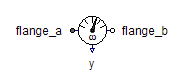Modulus Func - MapleSim Help

Modulus Func

Compute the modulusDescription The Modulus Func component computes the element-wise modulus over the reals. The Signal Size parameter assigns the dimension of the input and output connectors.
 Equations ${y}_{j}=\mathrm{mod}\left({u}_{j},\mathrm{md}\right)$

Connections

 Name Description Modelica ID $u$ Real input vector u $y$ Real output vector y

Parameters

 Name Default Units Description Modelica ID $\mathrm{md}$ $1$ Nonnegative number, the modulus md Signal Size $1$ Dimension of input and output signals signalSize

 Modelica Standard Library The component described in this topic is from the Modelica Standard Library. To view the original documentation, which includes author and copyright information, click here.